Rectangular Prisms
Cylinders
Triangular Prisms
Word Problems
100

What is the surface area of this prism?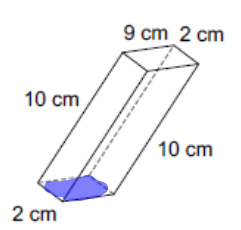256 cm^2

100

What shapes can be found in a cylinder when you unroll it into a "net"?

Two circles and a rectangle

100

Area of the base of this prism?120 m^2

100

Mr. Turner's classroom is 20 feet wide, 30 feet long, and 10 feet high. What is the surface area of his classroom?

2,200 ft2

200

What is the surface area of this prism?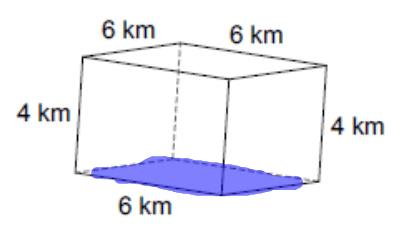192 km^2

200

What is the sum of the areas of the bases of this cylinder?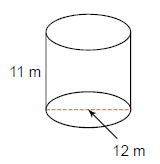226.2 m^2

200

Perimeter of the base of this prism?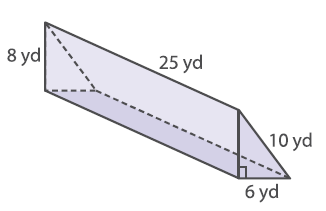24 yd

200

What is the surface area of a triangular prism with equilateral bases with sides of 10cm, height (triangle) of 8.7cm, and height (prism) of 15cm?

537 cm2

300

Total surface area of this prism?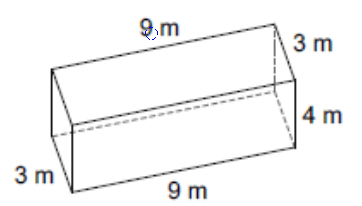150m^2

300

What is the total surface area of this cylinder?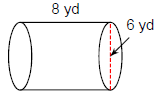207.3 yd^2

300

Lateral surface area of this prism?36 in

300

A cylindrical storage tank has a radius of 15 feet and a height of 30 feet. What is the surface area?

Approximately 4,241 ft2

400

Lateral surface area of this prism?220 cm^2

400

What is the Surface Area of the giant Coke Can?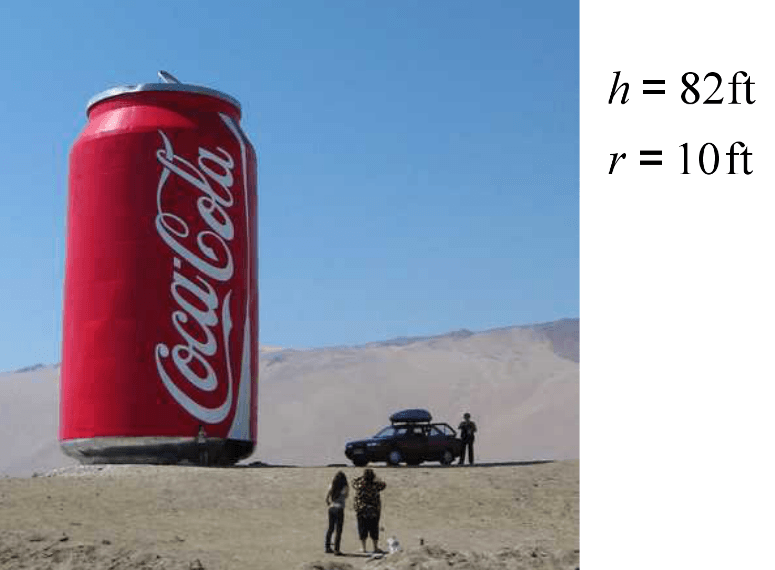5777.6 ft^2

400

Total surface area of this prism?191.4 mi^2

400

What is the surface area of a popcorn box with dimensions: 4in, 5in, and 10in tall.  The popcorn box has no top.

200 in2

500

1 gallon of paint covers 100 square feet.  How many gallons of paint would it take to cover the walls of a room that is 25 feet long, 30 feet wide, and 9 feet tall?

10 cans (you can't buy 9.9 cans)

500

What is the lateral surface area of Mr. Campbell's soup can which is 5 inches tall and has a radius of 3 inches?

94.2 inches squared

500

A tower is in the shape of a triangular prism. The bottom floor takes up 3,000 square feet. Each side of the tower is 750 feet long. The tower is 120 feet tall. What is the total surface area of the prism?

276,000 ft2

500

The dimensions of the recreation center floor are 150ft by 45ft, and the walls are 10 ft high. A gallon of paint will cover 400 square feet. About how much paint is needed to paint the walls of the recreation center?

3 gallons of paint

Click to zoom